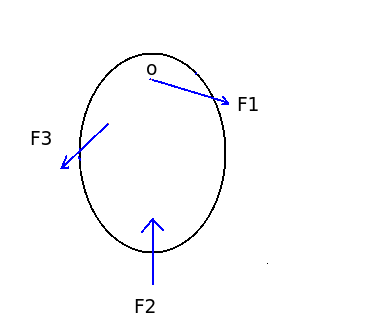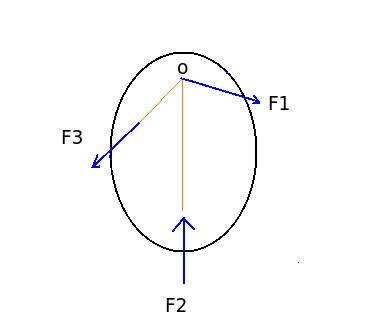# Line of action of forces on a body in equilibrium

Q.  If a body in equilibrium condition is acted by three forces at three points, then the line of action of these forces should be _____
- Published on 18 Sep 15

a. always concurrent
b. always parallel
c. concurrent or parallel
d. none of the above

#### Discussion

• Mohd azmal   -Posted on 23 Apr 17
• Sravanthi   -Posted on 16 Dec 15
- Different equilibrium conditions are to be satisfied when number of forces act together.- In the diagram shown above three forces are acting on an object. If summation of moment ΣM = 0 is the required condition, then third force must pass through the point where force F1 and F2 intersect.- Lami's theorem is a special condition of equilibrium when three forces act on an object.

- According to Lami's theorem, a body is in equilibrium condition if each force among three coplanar, concurrent forces are proportional to sine angle between other two.

## ➨ Post your comment / Share knowledgeEnter the code shown above:

(Note: If you cannot read the numbers in the above image, reload the page to generate a new one.)